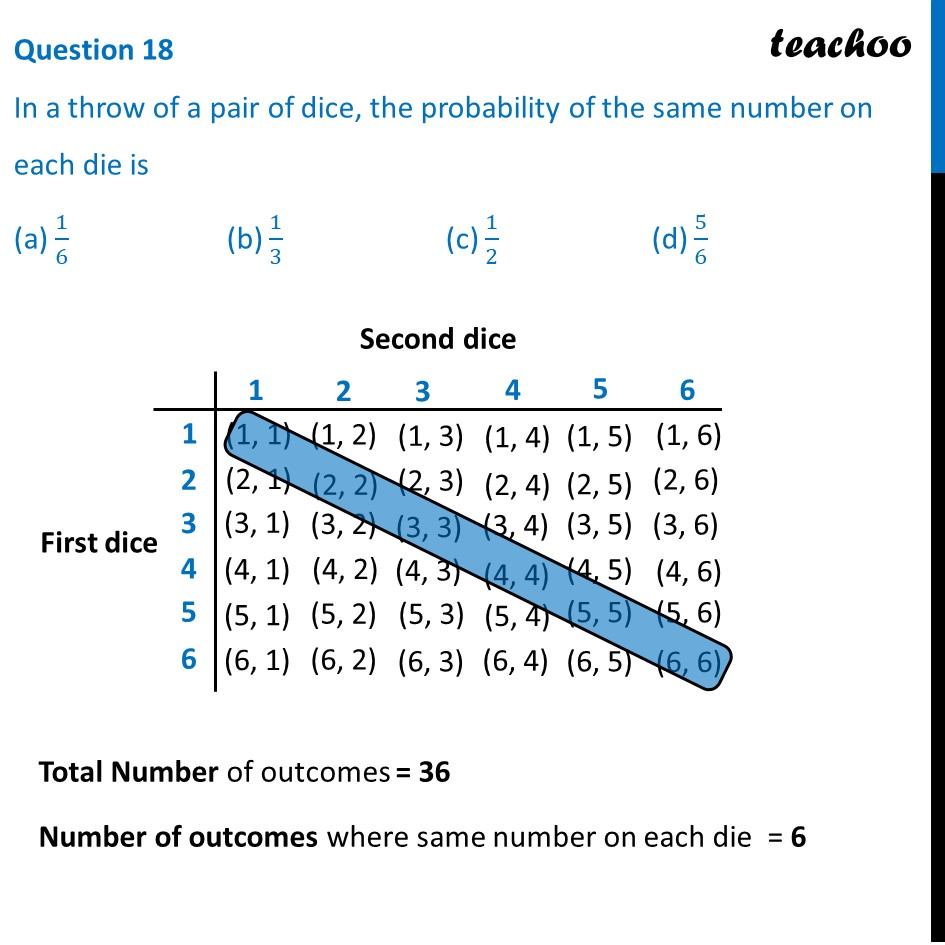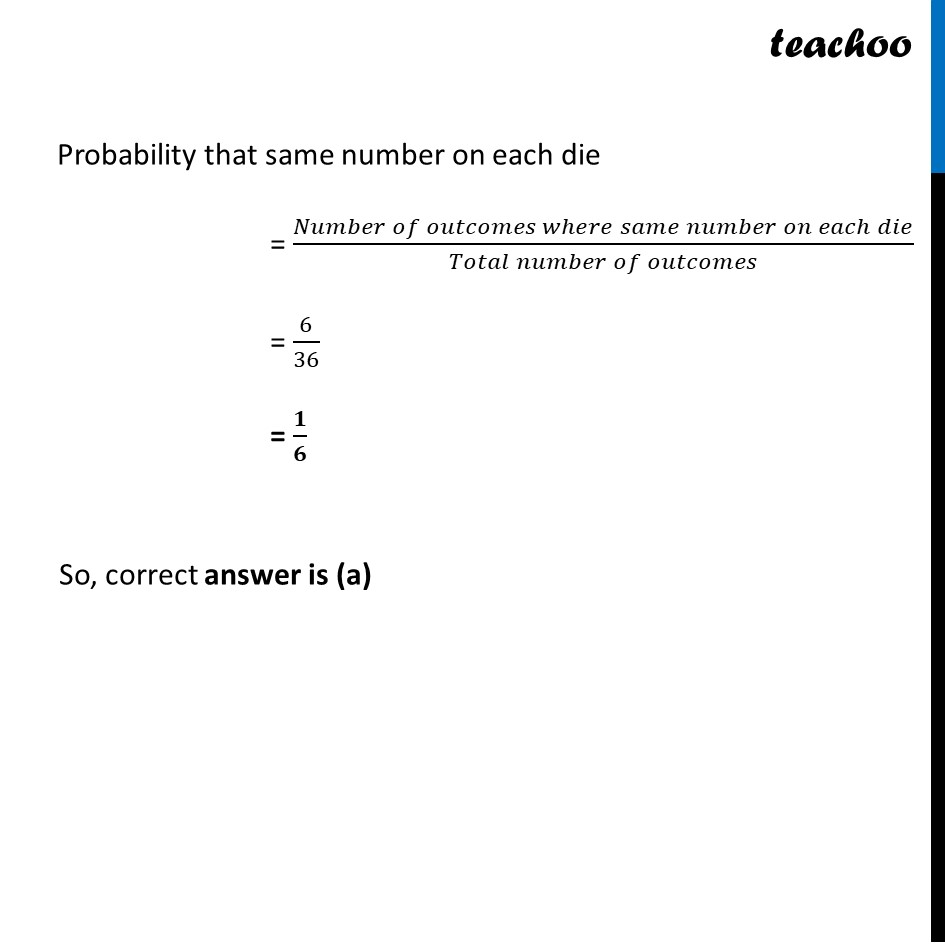CBSE Class 10 Sample Paper for 2022 Boards - Maths Basic [MCQ]

Class 10
Solutions of Sample Papers for Class 10 Boards

## (a) 1/6    (b) 1/3          (c) 1/2            (d) 5/6Get live Maths 1-on-1 Classs - Class 6 to 12

### Transcript

Question 18 In a throw of a pair of dice, the probability of the same number on each die is (a) 1/6 (b) 1/3 (c) 1/2 (d) 5/6 Total Number of outcomes = 36 Number of outcomes where same number on each die = 6 Probability that same number on each die = (𝑁𝑢𝑚𝑏𝑒𝑟 𝑜𝑓 𝑜𝑢𝑡𝑐𝑜𝑚𝑒𝑠 𝑤ℎ𝑒𝑟𝑒 𝑠𝑎𝑚𝑒 𝑛𝑢𝑚𝑏𝑒𝑟 𝑜𝑛 𝑒𝑎𝑐ℎ 𝑑𝑖𝑒)/(𝑇𝑜𝑡𝑎𝑙 𝑛𝑢𝑚𝑏𝑒𝑟 𝑜𝑓 𝑜𝑢𝑡𝑐𝑜𝑚𝑒𝑠) = 6/36 = 𝟏/𝟔 So, correct answer is (a)